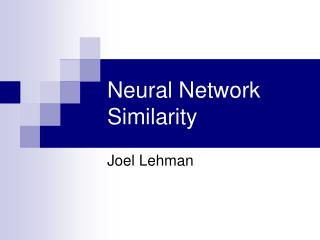DownloadDownload PresentationNeural Network Similarity

# Neural Network Similarity

Télécharger la présentation## Neural Network Similarity

- - - - - - - - - - - - - - - - - - - - - - - - - - - E N D - - - - - - - - - - - - - - - - - - - - - - - - - - -
##### Presentation Transcript

1. Neural Network Similarity Joel Lehman

2. Outline • Genetic Algorithms • Neural Networks • Evolving Neural Networks • Neural Network Similarity Problem • NP-Completeness Proof

3. Genetic Algorithms • Inspired by the apparent power of Natural Evolution • Usually applied to optimization problems • Consists of: • A population of candidate solutions • A mechanism for generating variation • A measure of goodness used for selection

4. Neural Networks • Computational abstraction of biological brains • Consist of nodes (neurons) and connections (synapses) • Can be drawn as a weighted digraph

5. Neural Networks

6. Evolving Neural Networks • Intelligence on earth evolved in form of biological brains • Using GA+NN is a very rough abstraction of how intelligence evolved • Can be used in many tasks • Robot control, game playing, classification, etc. • How to define genetic operators that operate on NN?

7. Evolving Neural Networks • Use a ‘direct encoding’ • Represent NN directly as a graph • Mutation is easy • Simply add new connections, nodes, or change connection weights • Crossover is hard • How to recognize what parts of one NN correspond to parts of another • Without this idea of ‘homology’ naïve crossover will likely just destroy the functionality of both NN

8. Neural Network Similarity Problem • Basically, question is: what parts of one NN are relatively the same to parts of a second NN • If we know this, we can intelligently do crossover to create meaningful offspring NN

9. Neural Network Similarity Problem • A Neural Network is composed of a 4-tuple: (Input Nodes, Output Nodes, Hidden Nodes, Connections) • Two NNs are ‘compatible’ if they share same Input and Output Nodes • A subnetwork of a neural network A is a NN compatible with A, with hidden nodes and connections that are subsets of those in A

10. Neural Network Similarity Problem • Given two compatible NNs, N1 and N2, an integer k, and a real number l • Does there exist a subnetwork NS1 of N1 with at least k connections isomorphic (disregarding connection weights) to a subnetwork NS2 of N2 such that the summed difference in connection weights between NS1 and NS2 are less than l.

11. NP Completeness Proof • First we must show it is in NP. • A witness can give the partial map between hidden nodes and connections in N1 to N2. It can then be verified in polynomial time that the conditions of k and l are satisfied

12. NP Completeness Proof • Basically this is an augmented version of subgraph isomorphism • Subgraph isomorphism • Given two graphs G1, G2, does there exist a subgraph of G1 with at least k edges that is isomorphic to a subgraph of G2?

13. NP Completeness Proof • Easy to map an instance of SGI into NNSP: • Create a NN from each graph by the following: • Set input and output nodes to null set • Vertices in the graph translate directly to hidden nodes • Edges in the graph translate directly to connections in the NN all with identical weights • K in NNSP is set to k from SGI • L in NNSP is set to 0 • Intuitively we know this works • We’ve stripped away all the augmentations

14. NP Completeness Proof • Given yes instance of SGI • The mapped instance of NNSP will also have an subgraph isomorphism, the NNs are isomorphic to the given graphs, and the L criterion is satisfied • Given yes instance of NNSP • We know there are no hidden/input nodes • All weights are 1.0 (and thus insignificant to L criterion) • The isomorphism between NN/Graph and the decision question means there must be a SGI of size k

15. Questions?